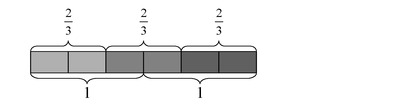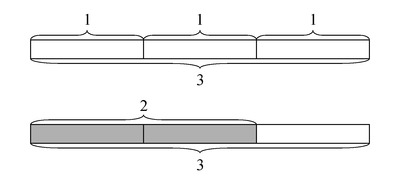# Connor and Makayla Discuss Multiplication

Alignments to Content Standards: 5.NF.B.4

Makayla said, "I can represent $3\times \frac23$ with 3 rectangles each of length $\frac23$."Connor said, “I know that $\frac23 \times 3$ can be thought of as $\frac23$ of 3. Is 3 copies of $\frac23$ the same as $\frac23$ of 3?”

1. Draw a diagram to represent $\frac23$ of 3.
2. Explain why your picture and Makayla’s picture together show that $3\times \frac23 = \frac23 \times 3$.
3. What property of multiplication do these pictures illustrate?

## IM Commentary

The purpose of this task is to

1. Have students think about the meaning of multiplying a whole number by a fraction, and
2. Compare this meaning to their previous understanding of multiplying a fraction by a whole number in order to see an instance of the commutative property of multiplication in the case of fractions.

Although the representations used in this task can be used to see the fact that $3 \times \frac23 = \frac23 \times 3$, it is not easy to use them to explain why the fact is true, or to see that multiplication is commutative for other numbers. This problem can be used to raise the question of why.

In grade 3, students saw why multiplication of whole numbers is commutative by looking at arrays or rectangles. After being introduced by this task to the idea that multiplication is also commutative for fractions, students should also look at rectangles with fractional side lengths to reason about why this is true.

Students should have some prior experience with interpreting $\frac{a}{b} \times q$ as as $a$ parts of a partition of $q$ into $b$ equal parts before they are ready to tackle parts (b) and (c) of the task.

Note that it is important to make sure the unit (the whole) is the same length in both diagrams. Keep an eye out for students who use a different length.

## Solution

1. A rectangle of length 3 can be built from three rectangles of length 1:Thus, $\frac23$ of the large rectangle is 2 of the smaller rectangles of length 1.
2. We can see in Makayla's picture that there are 3 groups of 2 thirds, so there are 6 thirds all together. Since 3 thirds is 1 whole, 6 thirds is $6\div3 =$ 2 wholes. So three rectangles each of length $\frac23$ has a total length of 2.In the picture for part (a), we can see that $\frac23$ of 3 is also 2. So this shows that 3 groups of $\frac23$ (an interpretation of $3\times \frac23$) is equal to $\frac23$ of 3 (an interpretation of $\frac23 \times 3$), or $$3\times \frac23 = \frac23 \times 3$$
3. These pictures illustrate the commutative property of multiplication.